Publicité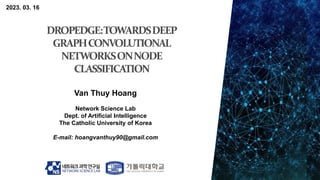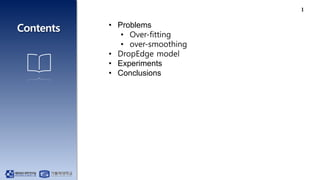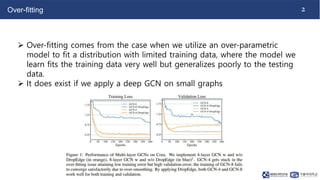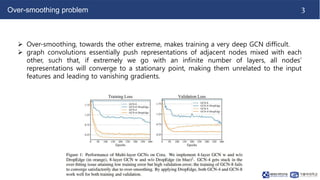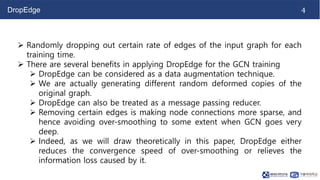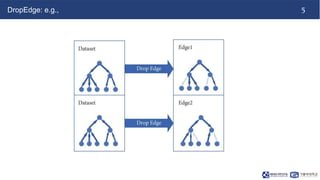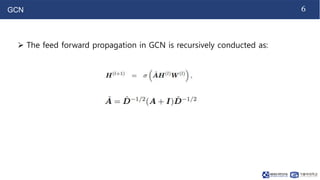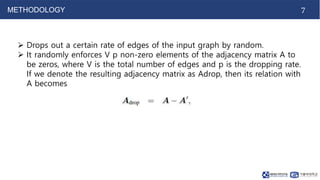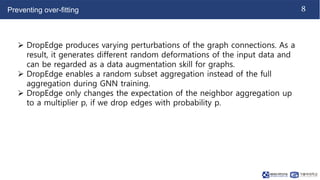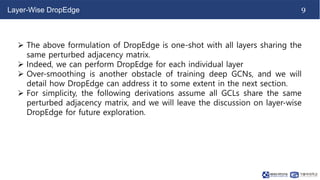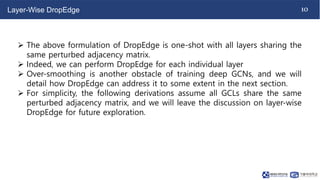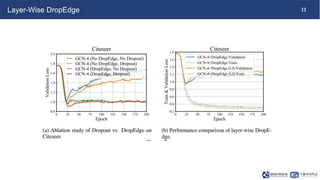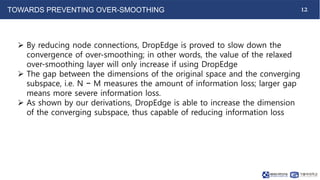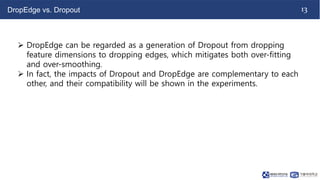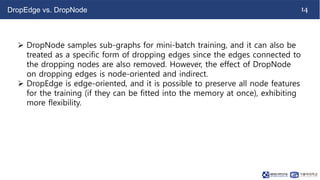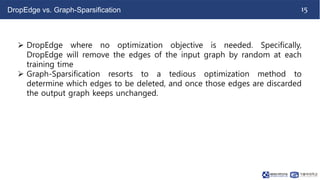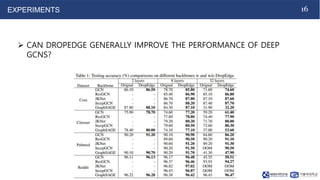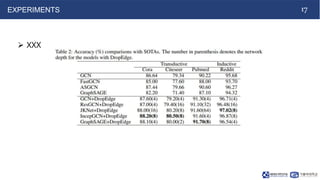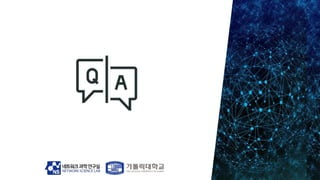1 sur 19
Publicité

### NS-CUK Seminar: V.T.Hoang, Review on "DropEdge: Towards Deep Graph Convolutional Networks on Node Classification", ICLR 2020

1. 2023. 03. 16 Van Thuy Hoang Network Science Lab Dept. of Artificial Intelligence The Catholic University of Korea E-mail: hoangvanthuy90@gmail.com
2. 1 • Problems • Over-fitting • over-smoothing • DropEdge model • Experiments • Conclusions
3. 2 Over-fitting  Over-fitting comes from the case when we utilize an over-parametric model to fit a distribution with limited training data, where the model we learn fits the training data very well but generalizes poorly to the testing data.  It does exist if we apply a deep GCN on small graphs
4. 3 Over-smoothing problem  Over-smoothing, towards the other extreme, makes training a very deep GCN difficult.  graph convolutions essentially push representations of adjacent nodes mixed with each other, such that, if extremely we go with an infinite number of layers, all nodes’ representations will converge to a stationary point, making them unrelated to the input features and leading to vanishing gradients.
5. 4 DropEdge  Randomly dropping out certain rate of edges of the input graph for each training time.  There are several benefits in applying DropEdge for the GCN training  DropEdge can be considered as a data augmentation technique.  We are actually generating different random deformed copies of the original graph.  DropEdge can also be treated as a message passing reducer.  Removing certain edges is making node connections more sparse, and hence avoiding over-smoothing to some extent when GCN goes very deep.  Indeed, as we will draw theoretically in this paper, DropEdge either reduces the convergence speed of over-smoothing or relieves the information loss caused by it.
6. 5 DropEdge: e.g.,
7. 6 GCN  The feed forward propagation in GCN is recursively conducted as:
8. 7 METHODOLOGY  Drops out a certain rate of edges of the input graph by random.  It randomly enforces V p non-zero elements of the adjacency matrix A to be zeros, where V is the total number of edges and p is the dropping rate. If we denote the resulting adjacency matrix as Adrop, then its relation with A becomes
9. 8 Preventing over-fitting  DropEdge produces varying perturbations of the graph connections. As a result, it generates different random deformations of the input data and can be regarded as a data augmentation skill for graphs.  DropEdge enables a random subset aggregation instead of the full aggregation during GNN training.  DropEdge only changes the expectation of the neighbor aggregation up to a multiplier p, if we drop edges with probability p.
10. 9 Layer-Wise DropEdge  The above formulation of DropEdge is one-shot with all layers sharing the same perturbed adjacency matrix.  Indeed, we can perform DropEdge for each individual layer  Over-smoothing is another obstacle of training deep GCNs, and we will detail how DropEdge can address it to some extent in the next section.  For simplicity, the following derivations assume all GCLs share the same perturbed adjacency matrix, and we will leave the discussion on layer-wise DropEdge for future exploration.
11. 10 Layer-Wise DropEdge  The above formulation of DropEdge is one-shot with all layers sharing the same perturbed adjacency matrix.  Indeed, we can perform DropEdge for each individual layer  Over-smoothing is another obstacle of training deep GCNs, and we will detail how DropEdge can address it to some extent in the next section.  For simplicity, the following derivations assume all GCLs share the same perturbed adjacency matrix, and we will leave the discussion on layer-wise DropEdge for future exploration.
12. 11 Layer-Wise DropEdge
13. 12 TOWARDS PREVENTING OVER-SMOOTHING  By reducing node connections, DropEdge is proved to slow down the convergence of over-smoothing; in other words, the value of the relaxed over-smoothing layer will only increase if using DropEdge  The gap between the dimensions of the original space and the converging subspace, i.e. N − M measures the amount of information loss; larger gap means more severe information loss.  As shown by our derivations, DropEdge is able to increase the dimension of the converging subspace, thus capable of reducing information loss
14. 13 DropEdge vs. Dropout  DropEdge can be regarded as a generation of Dropout from dropping feature dimensions to dropping edges, which mitigates both over-fitting and over-smoothing.  In fact, the impacts of Dropout and DropEdge are complementary to each other, and their compatibility will be shown in the experiments.
15. 14 DropEdge vs. DropNode  DropNode samples sub-graphs for mini-batch training, and it can also be treated as a specific form of dropping edges since the edges connected to the dropping nodes are also removed. However, the effect of DropNode on dropping edges is node-oriented and indirect.  DropEdge is edge-oriented, and it is possible to preserve all node features for the training (if they can be fitted into the memory at once), exhibiting more flexibility.
16. 15 DropEdge vs. Graph-Sparsification  DropEdge where no optimization objective is needed. Specifically, DropEdge will remove the edges of the input graph by random at each training time  Graph-Sparsification resorts to a tedious optimization method to determine which edges to be deleted, and once those edges are discarded the output graph keeps unchanged.
17. 16 EXPERIMENTS  CAN DROPEDGE GENERALLY IMPROVE THE PERFORMANCE OF DEEP GCNS?
18. 17 EXPERIMENTS  XXX
Publicité